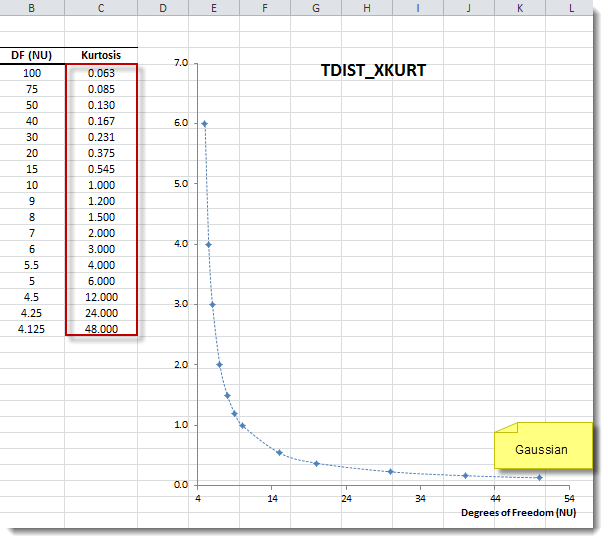# TDIST_XKURT - Kurtosis of t-distribution

Calculates the excess kurtosis of the Student's t-Distribution.

## Syntax

TDIST_XKURT(v)

v
is the degrees of freedom of the Student's t-Distribution (v > 4).

## Remarks

1. TDIST_XKURT is declared as deprecated. Please, use DIST_XKURT as TDIST_XKURT is listed here only for backward compatibility.
2. The probability density function of the Student's t-Distribution is defined as:
$$f(t) = \frac{\Gamma(\frac{\nu+1}{2})} {\sqrt{\nu\pi}\,\Gamma(\frac{\nu}{2})} \left(1+\frac{t^2}{\nu} \right)^{-(\nu+1)/2}$$ Where:
• $\Gamma (.)$ is the gamma function.
• $\nu$ is the degrees of freedom (i.e., shape parameter).
3. The excess kurtosis of t-Distribution is defined as: $$\gamma_2= \frac{6}{\nu-4}$$ Where:
• $\nu$ is the degrees of freedom.
4. IMPORTANT The Student's t-Distribution kurtosis is only defined for degrees of freedom values greater than 4.
5. Special Cases:
1. $\nu\to 4^+$. $$\lim_{\nu\to 4^+}\gamma_2(\nu)=+\infty$$
2. $\nu\to \infty$. $$\lim_{\nu\to +\infty}\gamma_2(\nu)=0$$

## Examples

Example 1:

Student's t-Distribution X-Kurtosis Plot:Example 2:

Formula Description (Result)
=TDIST_XKURT(5) Excess kurtosis with 5 degrees of freedom (6.000).
=TDIST_XKURT(100) Student t-dist approaches Normality as v >> 1 (0.063).
=TDIST_XKURT(4.002) Excess kurtosis increases as v approaches 4 (3000.000).